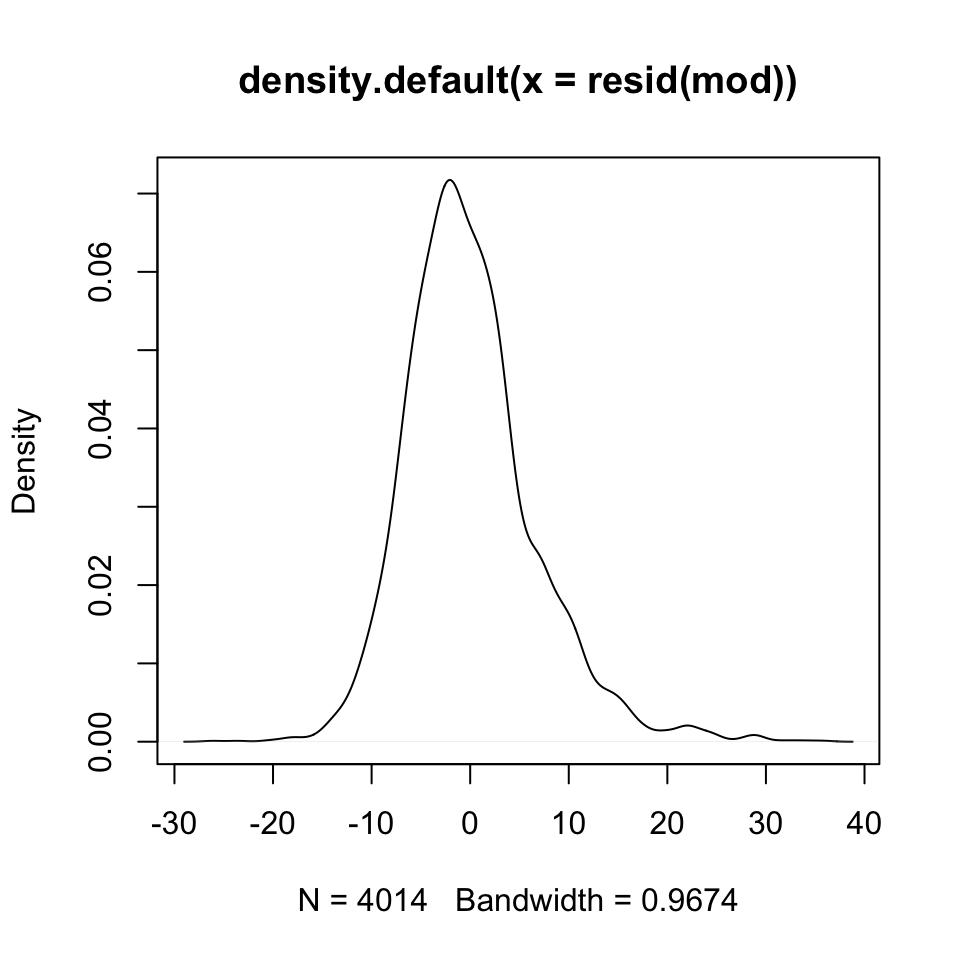This tutorial shows you:

• how to diagnose non-normally distributed errors
• how to diagnose heteroskedasticity
• how to diagnose collinearity
• how to transform variables
• how to obtain robust standard errors

Note on copying & pasting code from the PDF version of this tutorial: Please note that you may run into trouble if you copy & paste code from the PDF version of this tutorial into your R script. When the PDF is created, some characters (for instance, quotation marks or indentations) are converted into non-text characters that R won’t recognize. To use code from this tutorial, please type it yourself into your R script or you may copy & paste code from the source file for this tutorial which is posted on my website.

Note on R functions discussed in this tutorial: I don’t discuss many functions in detail here and therefore I encourage you to look up the help files for these functions or search the web for them before you use them. This will help you understand the functions better. Each of these functions is well-documented either in its help file (which you can access in R by typing ?ifelse, for instance) or on the web. The Companion to Applied Regression (see our syllabus) also provides many detailed explanations.

As always, please note that this tutorial only accompanies the other materials for Day 10 and that you are expected to have worked through the reading for that day before tackling this tutorial.

This week’s tutorial focuses on violations of the OLS assumptions. As a reminder, the assumptions you’ve encountered are:

• [A1] Linear relationships between each $$x$$ and $$y$$
• [A2] Constant error variance
• [A3] Normality of the errors: $$\varepsilon \sim \mathcal{N}(0, \sigma^2)$$
• [A4] Independence of observations
• [A5] No correlation b/w $$x$$ and $$\varepsilon$$: $$\text{cor}(x, \varepsilon) = 0$$

For violations of most of these assumptions, investigating the residuals of a regression model is a good start. Hence this tutorial will focus on residuals quite a bit. Residuals (or errors) are the differences between the observed values of $$y$$ and the predicted, or fitted, values for $$y$$, also known as $$\hat{y}$$:

$\varepsilon_i = y_i - \hat{y}_i$

You recall that we assume these residuals to be distributed normally with a mean of 0 and a constant variance. That is, the spread of the residuals around the predicted values is the same across the range of predicted values.

# Non-normally distributed errors

You read in section 12.1 of AR that non-normally distributed errors do not compromise the validity of OLS estimates in large samples, but they may give you a reason to improve your model. There are two simple graphical ways to diagnose whether the residuals are not normally distributed, and you’re already familiar with these methods from the tutorial for Day 3.

For an example, we’ll use data from the Canadian Survey of Labour and Income Dynamics, for the province of Ontario. Observations are individuals from Ontario who responded to the 1994 wave of the survey. These data are provided as part of the “car” package, but I uploaded them to my website as well. The following variables are included:

slid.dat <- rio::import("http://www.jkarreth.net/files/car_SLID.csv")
summary(slid.dat)
##      wages          education          age            sex
##  Min.   : 2.300   Min.   : 0.00   Min.   :16.00   Length:7425
##  1st Qu.: 9.235   1st Qu.:10.30   1st Qu.:30.00   Class :character
##  Median :14.090   Median :12.10   Median :41.00   Mode  :character
##  Mean   :15.553   Mean   :12.50   Mean   :43.98
##  3rd Qu.:19.800   3rd Qu.:14.53   3rd Qu.:57.00
##  Max.   :49.920   Max.   :20.00   Max.   :95.00
##  NA's   :3278     NA's   :249
##    language
##  Length:7425
##  Class :character
##  Mode  :character
##
##
##
## 

This dataset contains 5 variables:

Variable Description
wages Composite hourly wage rate from all jobs in Canadian dollars.
education Number of years of schooling.
age Age in years
sex A categorical variable: Female, Male.
language A categorical variable: English, French, Other.

As often before, we’d like to predict hourly wages (wages) with education and, in this case, other variables. To do so, we can fit an OLS regression model as usual:

mod <- lm(wages ~ education + age + sex, data = slid.dat)
summary(mod)
##
## Call:
## lm(formula = wages ~ education + age + sex, data = slid.dat)
##
## Residuals:
##     Min      1Q  Median      3Q     Max
## -26.111  -4.328  -0.792   3.243  35.892
##
## Coefficients:
##              Estimate Std. Error t value Pr(>|t|)
## (Intercept) -7.905243   0.607771  -13.01   <2e-16 ***
## education    0.918735   0.034514   26.62   <2e-16 ***
## age          0.255101   0.008634   29.55   <2e-16 ***
## sexMale      3.465251   0.208494   16.62   <2e-16 ***
## ---
## Signif. codes:  0 '***' 0.001 '**' 0.01 '*' 0.05 '.' 0.1 ' ' 1
##
## Residual standard error: 6.602 on 4010 degrees of freedom
##   (3411 observations deleted due to missingness)
## Multiple R-squared:  0.2972, Adjusted R-squared:  0.2967
## F-statistic: 565.3 on 3 and 4010 DF,  p-value: < 2.2e-16

To investigate the residuals of this regression, we can use the resid() or (equivalently) residuals() functions.

summary(resid(mod))
##     Min.  1st Qu.   Median     Mean  3rd Qu.     Max.
## -26.1100  -4.3280  -0.7925   0.0000   3.2430  35.8900

But these quantities don’t tell us much about the distribution of the residuals.

## Density plot

Hence, a density plot is often useful. You’re already familiar with density plots (and histograms) from Day 3.

plot(density(resid(mod)))Last week (Day 9), you learned that normalizing/standardizing residuals often is useful because studentized residuals because they follow a t-distribution. You can obtain the studentized residuals using the rstudent() function.

summary(rstudent(mod))
##      Min.   1st Qu.    Median      Mean   3rd Qu.      Max.
## -3.968000 -0.656000 -0.120100  0.000099  0.491500  5.460000
plot(density(rstudent(mod)))